﻿ A Simulation Showing the Role of Central Limit Theorem in Handling Non-Normal DistributionsPublications are Open
Access in this journal
Article Versions
Export Article
• Normal Style
• MLA Style
• APA Style
• Chicago Style
Research Article
Open Access Peer-reviewed

### A Simulation Showing the Role of Central Limit Theorem in Handling Non-Normal Distributions

Moatasim A. Barri
American Journal of Educational Research. 2019, 7(8), 591-598. DOI: 10.12691/education-7-8-8
Received June 28, 2019; Revised August 14, 2019; Accepted August 23, 2019

### Abstract

This simulation employed a compiler which explains the role of central limit theorem in dealing with populations that are not normally distributed. A group of 10000-data-point populations were simulated according to five different kinds of distribution: uniform, platykurtic normal, positively-skewed exponential, negatively-skewed triangular, and bimodal. Three 500-data-point sampling distributions of sample sizes of 2, 10, and 30 were created from each population. All populations and sampling distributions were displayed in histograms for analysis along with their means and standard deviations. The results verified the principles of the central limit theorem and indicated that if the population is close to normality, a smaller sample size is needed so that the central limit theorem can take effect. But if the population is far from normality, a large sample size might be required. A proportion of population was proposed for a sample size based on the simulation results. Further studies and implications are discussed.

### 1. Introduction

People all over the world were created in different heights and widths. Let’s say that hundreds of millions of men and women get together in one location such as the United States. It would be impossible to tell in advance what the height of an individual chosen from the U.S. would be. Obviously, something is needed to serve as a clear and convenient synopsis of the heights of all people living in the U.S. That could be attained by what are called statistics.

The importance of statistics appears when researchers attempt to answer this kind of question: what is the true average length of people living in, for example, the U.S.? The first step for answering this question is to collect data relating to the lengths of American people. However, do all people in the U. S. have to be considered, so the true average of their lengths could be obtained? It is almost always impossible to place all American people in a line and get their lengths with perfect measurements. So, the practical solution here is to randomly select a representative sample from the American population and then collect the lengths of all individuals included in that sample. The population must be clearly specified; otherwise the sample will be poorly drawn from the population 13.

Once data are collected, the second step is to decide on an appropriate measure which can describe the lengths of American people. There are many possible measures to choose from such as the mean, median or mode. Each is calculated in a different way and explains the data from a different point of view and can characterize the average length in a numeric quantity. Among these measures the mean will be considered since it is one of the most useful and widely used. It is the value that represents the center of gravity or the balance point of the distribution 6.

The sample mean is generally used as an estimator of the population mean. When the expected value of the sample mean is similar to the population mean, researchers can draw generalizations from the sample to the population. That is the essential role of inferential statistics. The term statistic is generally used to describe a sample while the term parameter is used to describe a population. Statistics are almost always used since the perfect values of parameters are unknown. Particularly when the population size is enormous, like the American population, there is no way to get perfect measurements for all subjects.

From one sample to the next, how do researchers guarantee that the values of the sample means are not varied? Let’s suppose that the researchers select two random samples and calculate their means. They may find that the second mean is considerably different from the first. According to its nature, random sampling can sometimes yield unexpected results even when perfectly administered. The difference between the two means might occur because the first sample could be made up mostly of tall individuals while the second could be made up of short ones. To prevent these variations in sample means, the researchers must believe in the central limit theorem that calls for using the law of large numbers 2 when choosing a sample size. A reasonable large sample could never be composed entirely of either tall or short individuals. For instance, flipping a coin a thousand of times will show up heads in almost 50% of the flips while flipping it three times might never show up heads 50% of the time.

However, how do researchers guarantee that a random large sample mean is close to a population mean? Having faith in the central limit theorem, the researchers can be sure that a mean of any reasonable large sample drawn randomly from a population will be close to the true mean of that population 11. The central limit theorem is the theorem that specifies the nature of the sampling distribution of means according to the central tendency, the variability, and the shape of the distribution 3. The sampling distribution of means is defined as the frequency distribution of means for all of the possible equal-sized samples drawn randomly from a given population 14. All the important properties of the sampling distribution can be summed up in the central limit theorem 4. According to 7, the sampling distribution has the following properties: (a) the mean of the sampling distribution is always equal to the mean of the population; (b) the sampling distribution also tends to be less variable than the population; and (c) the shape of the sampling distribution starts looking like a normal distribution, even when the population is not normally distributed. Any change in a sample size can cause a change in the shape of the sampling distribution of means 10. What proportion of population does fit reasonably a sample size, so the sample distribution is going to be normal and its mean will be close to the true mean of the population?

One of the most common concerns in research is the calculation of an effective sample size. The larger the sample size is, the more accurate the study results would be. The results from an effective sample size would be valid, while the results from an inappropriate size would be doubtful. This simulation had a twofold purpose and utilized a compiler: (a) to concretely reveal the role of the central limit theorem in handling non-normal populations and (b) to propose a population proportion for a sample size so the central limit theorem can take effect despite the distance of population distribution from normality.

### 2. Distributions

2.1. Normal Distribution

The normal distribution is usually characterized as a mathematical bell-shaped curve with one peak (see Figure 1). It is symmetric around its mean and never touches the horizontal axis. The mean, mod, and median values of the normal distribution are all the same and are located at the center of the distribution.

The normal distribution is controlled by two quantities: (a) the mean where a single peak of the distribution occurs and (b) the standard deviation which indicates the extent of dispersion for the distribution as a whole 1. This means that different quantities of the mean and standard deviation produce different styles of normal distributions 9 as follows:

1. Platykurtic that tends to be flat (see Figure 2A),

2. Leptokurtic that tends to be so peaked (see Figure 2B), and

3. Mesokurtic that falls in between and looks as in Figure 2C.

• Figure 1. The normal distribution
• Figure 2A. Platykurtic
• Figure 2B. Leptokurtic

Even though there are many styles of bell-shaped curves, they all possess a set of properties that characterize them in a uniform manner, including:

(1) Unimodality, which refers to possessing a single mode that falls exactly at the center of the distribution,

(2) Symmetry, where the line of symmetry is perpendicular to the abscissa and falls exactly at the center of the distribution,

(3) Equality of descriptive statistics such as mean, mode, and median, where all fall at the center of the normal distribution, and

(4) Asymptote, where the normal distribution never touches the abscissa.

The normal distribution is defined by probability density function as follows:where P(x) is the height of the distribution at any value of x, x is an observed score,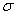is the standard deviation of distribution, andis the mean of distribution.

The normal distribution, with a mean of 0 and a standard deviation of 1, is often referred to as the standard normal distribution (see Figure 3). The probability density function for the standard normal distribution is as follows: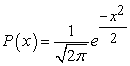• Figure 3. The standard normal distribution
2.2. Skewed Distribution

Any distribution that has a tail longer than the other is called skewed. If the longer tail is placed on the right, the distribution is positively skewed, sometimes called right-skewed (see Figure 4A). This means that most of the values in the distribution have a tendency to the left. When students were administered a very difficult test and most of them did poorly on it, their scores on the test would most likely follow a positively skewed distribution. If the longer tail of a distribution is placed on the left, the distribution in this case is negatively skewed, sometimes called left-skewed (see Figure 4B). This means that most of the values in the data have a tendency to the right. When students were administered a very easy test and most of them did very well on it, their scores on the test would most likely follow a negatively skewed distribution.

• Figure 4A. Positively-skewed distribution
• Figure 4B. Negatively-skewed distribution

Measures of central tendency, such as mean, median, and mode, are influenced by skewness of the distribution. In the case of the negatively-skewed distribution, the mean is less than the median, which is, in turn, less than the mode. If the distribution is skewed to the right, the mean is greater than the median, which is, in turn, greater than the mode. In the absence of graphing, it could be possible to identify whether the data are skewed to the left or to the right by obtaining the mean and median values of the data and using the following two rules: (a) if the mean is much larger than the median, the data would be skewed to the right, (b) if the mean is much smaller than the median, the data would be skewed to the left.

There are many kinds of skewed distributions including triangular and exponential distributions, each of which is explained briefly in the following section.

2.2.1. Exponential Distribution

The exponential distribution is a positively-skewed distribution as shown in a Figure 5 with a probability density function defined as follows: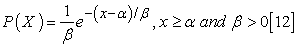where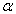is the location parameter which simply translates the graph to the left or the right on the horizontal axis and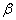is the scale parameter which stretches out the graph. If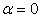andtheforand is called the standard exponential distribution. Figure 5, for example, shows the probability density function of an exponential distribution withand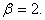• Figure 5. Positively-skewed exponential distribution

2.2.2. Triangular Distribution

The triangular distribution is a continuous distribution with a probability density function shaped like a triangle (see Figure 6A & Figure 6B) and defined as follows: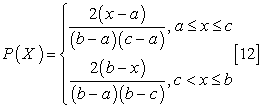where a is the minimum value, b is the maximum value, and c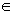[a, b] and is the peak value (the mode). The maximum value of the probability density function isand occurs at c. Figure 6A and Figure 6B, for example, show the probability density function of triangular distributions with a = 0, b = 10, and a maximum value of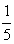at c = 9.5 (see Figure 6A) and c = 0.5 (see Figure 6B).

• Figure 6A. Negatively-skewed triangular distribution
• Figure 6B. Positively-skewed triangular distribution
2.3. Uniform Distribution

It is a distribution where any value in a range defined by the minimum and maximum values has equal probability of occurrence (see Figure 7). The rectangular distribution is a sign that the distribution is not normal.

• Figure 7. Uniform distribution

The probability density function of the uniform distribution is as follows: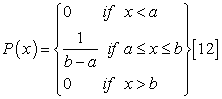where a is the minimum value and b is the maximum value (see Figure 8).

• Figure 8. The uniform distribution with a range of [a, b]
2.4. Multimodal Distribution

Any distribution that has more than one mode is referred to as a multimodal distribution. If the distribution has two modes or two relative peaks, it is called bimodal (see Figure 9A). The distribution which has three modes or three relative peaks is called trimodal (see Figure 9B). Multimodality of the distribution is a strong sign that the distribution is not normal. The multimodality indicates that the distribution is heterogeneous, meaning that the distribution is, in fact, derived from two or more distributions which have things in common.

• Figure 9A. Bimodal distribution
• Figure 9B. Trimodal distribution

### 3. The Advantage of the Central Limit Theorem

In fact, collecting all data from a whole population is not a practical way, so statistics are used instead by sampling the population randomly and then drawing inferences from this sample to the whole population. However, what happens if the population per se is not normally distributed? Even if the population is normally distributed, how do you guarantee that, from one sample to the next, the values of the sample means are not varied? To answer these questions, you must have faith in the central limit theorem, which implies selecting large random samples from the population and calculating the mean for each sample. This generates the distribution of the sample means, which, in most cases, follows the normal curve and confirms the idea that the mean of any sample drawn from the population is close to the true mean of the population.

As stated by 5, the central limit theorem contains three principles as follows:

(1) A sampling distribution looks more and more normal as the sample size is increased, even when the population distribution itself is not normal.

(2) Regardless of the population distribution, the variability of a sampling distribution, as measured by the standard deviation, decreases as the sample size is increased.

(3) Regardless of the population distribution and sample size, a sampling distribution always has a mean equal to the mean of the population from which it is drawn.

### 4. Method

This simulation was quantitative in nature and employed a Fortran-95-language-based compiler for data generation and analysis.

4.1. The Central Limit Theorem Compiler

A compiler was developed by the author using the FTN95: Fortran 8 to: (a) confirm the principles according to which the central limit theorem functions and (b) to propose a population proportion for a sample size so the central limit theorem can take effect despite the distance of population distribution from normality. The compiler generates data according to multiple kinds of distributions including normal, right-skewed triangular, left-skewed triangular, uniform, exponential, bimodal and multimodal; creates histograms; and calculates mean, standard deviation, maximum and minimum values of distributions.

4.2. Basic Codes for Data Generation

The basic style codes for generating a number of random data points following a given distribution are explained in the following section.

4.2.1. The Normal Distribution Code

The basic style code for generating the normal distribution is mentioned below: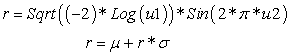where u1 and u2 are random real numbers, and μ and σ are constants that represent the mean and standard deviation of the normal distribution respectively.

4.2.2. The Uniform Distribution Code

The basic style code mentioned below is employed to generate the uniform distribution.where u is a random real number and min and max are constants that represent the lower and upper bounds of the uniform distribution respectively.

4.2.3. The Triangular Distribution Code

The following basic style code is used for generating the triangular distribution:

If (u <= (mode-min)/(max-min)) Then

r = min + sqrt (u * (max-min) * (mode-min))

Else If (u > (mode-min)/(max-min)) then

r = max – sqrt ((1-u) *(max-min)*(max-mode))

End If

where max and min are constants that represent the upper and lower bounds of a triangular distribution, u is a random real number, and mode is a modal value of the triangular distribution which is usually set subjectively and works as a determinant whether the distribution is positively- or negatively-skewed based on the following rules:

(1) If the mode value equals or is close to the minimum value, the code will generate a positively skewed distribution.

(2) If the mode value equals or is close to the maximum value, the code will generate a negatively skewed distribution.

4.2.4. The Exponential Distribution Code

The following code is utilized to generate the exponential distribution.whereis the location parameter,is the scale parameter, and u is a random positive real number.

4.2.5. Multimodal Distribution Code

The basic code for generating random data points following a normal distribution, shown previously, can be used two times to create a distribution with two modes or multiple times to create a distribution with multiple modes. Each time the code is applied, the mean value, at least, must be different.

4.3. Testing Conditions

To explain how the central limit theorem works, the central limit theorem compiler was used to simulate a group of 10,000 data-point populations and sampling distributions of sample sizes of 2, 10, and 30, each of which was drawn 500 times according to five different kinds of distributed population as follows:

(1) A uniformly distributed population which had two parameters, the lower bound, 0, and the upper bound, 10;

(2) A platykurtic normal population which has two parameters, mean of 5, and standard deviation of 2.5;

(3) A positively-skewed population (e.g., an exponential distribution with a scale parameter of 2 and a location parameter of 1;

(4) A negatively-skewed population (e.g., a triangular distribution with a minimum value of 0, a maximum value of 10, and a mode value of 9.5); and

(5) A bimodal population which has five parameters: the proportion of values or “contribution” from the first and second distributions (0.5), the mean of the first distribution (0), the standard deviation of the first distribution (1), the mean of the second distribution (10), and the standard deviation of the second distribution (1).

4.4. Data Analysis

Three common characteristics were considered to examine population and sampling distributions including the shape, central tendency, and variability. The histogram was considered since it is the most common statistical graph that can describe the shape of a frequency distribution in a clear fashion. The Statistical Package for Social Sciences (SPSS) was used for creating histograms for population and sampling distributions. Each population distribution was displayed in a histogram along with the three sampling distributions of sample sizes of 2, 10, and 30, each of which was displayed in a separate histogram.

The mean was used to measure the central tendency of population and sampling distributions. Although the mean is useful in identifying the center of statistical data, it delivers only part of the story and fails to deliver the other interesting part. Actually, the mean cannot tell researchers whether the data are close to its center or whether the data are spread out over a wide range. However, a measure like the standard deviation can tell that missing part! Accordingly, the standard deviation was computed for population and sampling distributions for complete analysis.

### 5. Results and Discussion

A sampling distribution dragged from a uniform population (see Figure 10A) starts approaching the normal distribution even when the sample size is small (see Figure 10B). As the sample size increases to 10, the sampling distribution tends to be closer to the normal distribution (see Figure 10C). With a sample size of 30, the sampling distribution tends to be a typical normal curve (see Figure 10D). As the sample size increases, the variability of each sampling distribution decreases while the mean is still adjacent to the true mean of the population.

• Figure 10A. Histogram of population–uniform distribution: population = 10,000; mean = 5.03; standard deviation = 2.88
• Figure 10B. Sampling distribution of means (from a uniform distribution): sample size = 2; number of samples = 500; mean = 4.92; standard deviation = 2.048
• Figure 10C. Sampling distribution of means (from a uniform distribution): sample size = 10: number of samples = 500; mean = 4.94; standard deviation = 0.94
• Figure 10D. Sampling distribution of means (from a uniform distribution): sample size = 30; number of samples = 500; mean = 5.05; standard deviation = 0.51

Figure 11A shows a platykurtic normal population that tends to be flat and broad. Sampling distributions dragged from the platykurtic normal distribution start approaching a perfect normal curve (mesokurtic) and tend to be less variable as the sample size increases (see Figures 11B and 11C). With a large enough sample size, the variability of the sampling distribution drops considerably and the sampling distribution starts looking leptokurtic where the sampling distribution is too peaked to be normal (see Figure 11D).

• Figure 11A. Histogram of population – platykurtic normal distribution: population = 10,000; mean = 5.00; standard deviation = 2.50
• Figure 11B. Sampling distribution of means (from a platykurtic normal distribution): sample size = 2; number of samples = 500; mean = 5.24; standard deviation = 1.83
• Figure 11C. Sampling distribution of means (from a platykurtic normal distribution): sample size = 10; number of samples = 500; mean = 5.00; standard deviation = 0.82
• Figure 11D. Sampling distribution of means (from a platykurtic normal distribution): sample size = 30; number of samples = 500; mean = 5.00; standard deviation= 0.43

The central limit theorem works well with the platykurtic normal and uniform populations when a sample size is small (n = 2). However, in the case of the positively and negatively skewed populations (see Figure 12A and Figure 13A), the central limit theorem requires taking more than the sample size of 2, so that the sampling distributions can meet the normality principle. For example, the sampling distribution shown in Figure 12B is still skewed to the right, while the sampling distribution shown in Figure 13B is to the left. When the sample size is increased to 10 (see Figure 12C and Figure 13C), both the sampling distributions get away from skewness and start to have a similar appearance to the normal distribution. With a large enough sample size, the sampling distributions displayed in Figure 12D and Figure 13D become less variable and follow the properties of the normal distribution and their means are close to the perfect mean of their populations.

• Figure 12A. Histogram of population –positively-skewed exponential distribution: population = 10,000; mean = 1.98; standard deviation = 1.98
• Figure 12B. Sampling distribution of means (from positively-skewed exponential distribution): sample size = 2; number of samples = 500; mean = 2.01; standard deviation = 1.45
• Figure 12C. Sampling distribution of means (from positively-skewed exponential distribution):sample size =10; number of samples = 500; mean = 1.97; standard deviation = 0.62
• Figure 12D. Sampling distribution of means (from positively-skewed exponential distribution):sample size =30; number of samples = 500; mean = 2.00; standard deviation = 0.39
• Figure 13A. Histogram of population–negatively-skewed triangular distribution: population = 10,000; mean = 6.53; standard deviation = 2.29
• Figure 13B. Sampling distribution of means (from a negatively skewed triangular distribution): sample size = 2; number of samples = 500; mean = 6.44; standard deviation = 1.56
• Figure 13C. Sampling distribution of means (from a negatively-skewed triangular distribution): sample size = 10; number of samples = 500; mean = 6.45; standard deviation = 0.76
• Figure 13D. Sampling distribution of means (from a negatively-skewed triangular distribution): sample size = 30: number of samples = 500; mean = 6.54; standard deviation = 0.40

In the case of the bimodal population (see Figure 14A), the central limit theorem needs to take more than the sample size of 2 to do its job perfectly in terms of normality. For instance, the sampling bimodal distribution shown in Figure 14B does not meet the normality principle with a sample size of 2, due to the resulting multi-peaks. However, by increasing the sample size to 10 (see Figure 14C), the multi-peak case completely disappeared and the resulting sampling distribution is prone to the properties of the normal distribution in terms of symmetry and unimodality. Eventually, with a large sample size, the sampling distribution starts to resemble a perfect normal distribution (see Figure 14D). It is also noticed that an increase in the sample size follows a decrease in the variability of the sampling distribution, while the mean value is restored to the true mean of the population.

• Figure 14A. Histogram of population –bimodal distribution: population = 10,000; mean = 5.00; standard deviation = 5.10
• Figure 14B. Sampling distribution of means (from a bimodal distribution): sample size = 2; number of samples = 500; mean = 4.79; standard deviation = 3.57
• Figure 14C. Sampling distribution of means (from a bimodal distribution): sample size = 10; number of samples = 500; mean = 5.04; standard deviation = 1.64
• Figure 14D. Sampling distribution of means (from a bimodal distribution): sample size = 30; number of samples = 500; mean = 5.02; standard deviation = 0.92

### 6. Conclusion

The results discussed previously confirmed the principles held by the central limit theorem. As the sample size is increased, (a) the variability of the sampling distribution decreases; (b) the sampling distribution increasingly approaches a normal distribution regardless of the shape of the population; and (c) the mean of the sampling distribution always has a mean equal to the mean of the population from which it is drawn.

In virtue of the central limit theorem, researchers can be sure that the mean of one reasonably large randomly chosen sample, regardless of the size and the shape of the population, will be close to the perfect mean of the intended population. If the researchers need more sureness, they need only to increase the sample size. How large a sample size is needed so the central limit theorem can take effect? Generally, the closer the population is to normality, a smaller sample size is needed to demonstrate the central limit theorem. If populations are heavily skewed or have several modes, they might require larger sample sizes. There is no certain rule of thumb to determine an appropriate sample size. Based on the simulation results, a ratio of 3:1000 could be proposed for a sample size, so the central limit theorem can become effective and inferences could be drawn despite the distance of population from normality.

In this simulation, researchers can see and feel the wonderful advantage of the central limit theorem. If it were not available at this moment, it would be impossible to use a statistic for estimating a parameter by using an average resulting from a reasonably large randomly chosen sample. In fact, the central limit theorem is the reason that research in social sciences and evaluation of new medications are still in existence. As with any Monte Carlo investigation, only a restricted number of factors could be inspected, so care must be considered when any generalization is made to other testing conditions. Further simulation investigations are needed to determine an appropriate sample size according to confidence intervals, effect size, power, the probabilities of Type I and II errors, and the number of independent variables.

### References

  Cohen, B. H. and Lea, R. B, Essentials of statistics for the social and behavioral sciences, John Wiley & Sons Inc, Hoboken, (2004). In article  Feller, W, An introduction to probability theory and its applications, Wiley, New York, (1970). In article  Heiman, G. W, Basic statistics for the behavioral sciences, Wadsworth Cengage Learning, Belmont, (2014). In article  Howell, D. C, Fundamental statistics for the behavioral sciences, Wadsworth Cengage Learning, Belmont, (2011). In article  Keppel, G. and Wickens, T. D, Design and analysis: A researcher's handbook, Prentice Hall, Upper Saddle River, (2004). In article  Paret, M. and Martz, E, “Understanding the Central Limit Theorem: Tumbling dice and birthdays,” (2009). [online]. Available: http://www.qualitydigest.com/inside/twitter-ed/understanding-central-limit-theorem.html In article  Pagano, R, Understanding statistics in the behavioral sciences, Wadsworth Cengage Learning, Belmont, (2013). In article  Silverfrost, "FTN95: Fortran 95 for Windows," (2012). [online]. Available:http://www.silverfrost.com/11/ftn95_overview.aspx In article  Shavelson, R. J, Statistical reasoning for the behavioral sciences, Allyn and Bacon, Boston, (1996). In article  Thompson, B, Foundations of behavioral statistics: An insight-based approach, Guilford Press, New York, (2006). In article  Wallnau, L. and Gravetter, F, Essentials of statistics for the behavioral sciences, Wadsworth Cengage Learning, Belmont, (2014). In article  Weisstein, E. W, "Normal Distribution," (2012). [online]. Available: http://mathworld.wolfram.com/NormalDistribution.html. In article  Welkowitz, J., Cohen, B. H. and Lea R. B, Introductory statistics for the behavioral sciences, John Wiley & Sons Inc, Hoboken, (2012). In article  Nolan, S. A. and Heinzen, T, Statistics for the Behavioral Sciences, Worth Publishers, New York, (2012). In articleThis work is licensed under a Creative Commons Attribution 4.0 International License. To view a copy of this license, visit http://creativecommons.org/licenses/by/4.0/